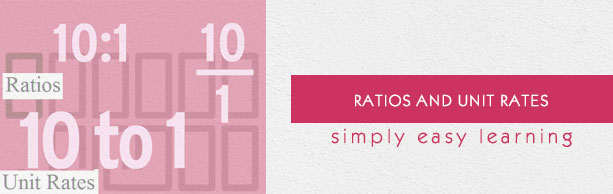# Ratios and Unit Rates

This tutorial provides comprehensive coverage of ratios and unit rates based on Common Core (CCSS) and State Standards and its prerequisites. Students can navigate learning paths based on their level of readiness. Institutional users may customize the scope and sequence to meet curricular needs. This simple tutorial uses appropriate examples to help you understand ratios and unit rates in a general and quick way.

# Audience

This tutorial has been prepared for beginners to help them understand the basics of ratios and unit rates. After completing this tutorial, you will find yourself at a moderate level of expertise in ratios and unit rates, from where you can advance further.

# Prerequisites

Before proceeding with this tutorial, you need a basic knowledge of elementary math concepts such as number sense, addition, subtraction, multiplication, division, whole numbers, fractions, types of fractions, decimals, comparing and ordering whole numbers and so on.